﻿ Our response surface technology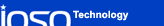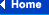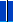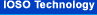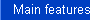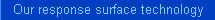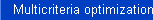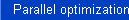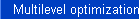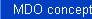### Response surface technology  in details

In IOSO Technology algorithms each iteration consists of two steps:

• constructing response surface approximations for objective function and constraints;
• optimizing the response surface approximations.

When moving from one iteration to the next we use special moving strategy that adapts algorithm parameters and structure for the particular optimization problem.

Several highly efficient algorithms are used to construct the response surface approximations:

##### Adaptive algorithms of regression analysis

Adaptive algorithms of regression analysis are based on modified least squares method. These algorithms adaptively define basis functions, parameters, and structure of such a regression function that provides the best approximation properties while requiring minimal number of points in the experiment plan. Such algorithms are successfully used in optimization problems of small and moderate dimensionality.

Some examples of adaptive algorithms application:

 Original function Responce surface approximation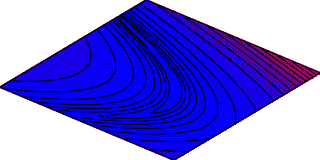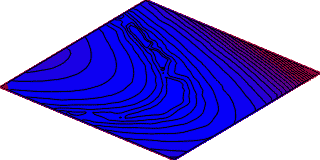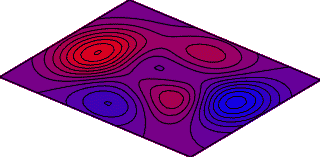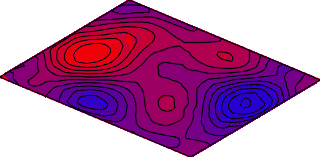##### Evolutionary self-organizing algorithms

The unique properties of the evolutionary self-organizing algorithms allow constructing response surface approximations with high predictive capabilities for the objective functions dependent on the large number of design variables (hundreds). Such function can potentially have very complex topology. However, the number of points required to construct such approximations using evolutionary self-organizing algorithms is minimal (30-40).

The evolutionary self-organizing algorithms are based on the modified version of the Method of Accounting for the Groups of Arguments.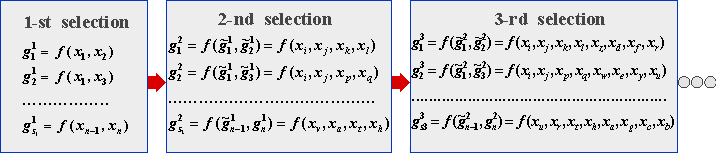##### Approximation process scheme.

Such algorithms employ the evolutionary procedure of constructing approximation function in the form of multilevel graph, solving structure-parametric approximation problem in the process.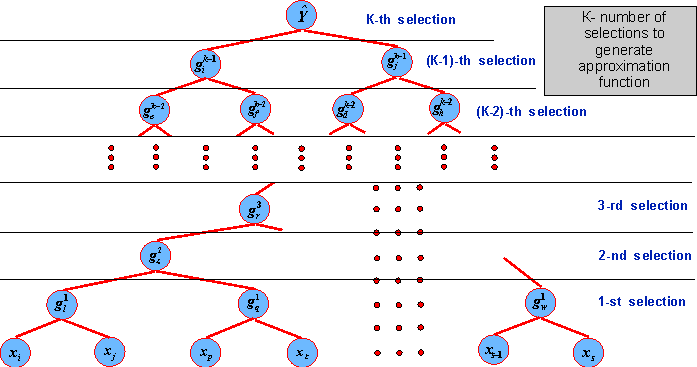Example of the response surface structure.
##### Neural network algorithms

The distinct feature of the developed neural network algorithms for constructing the responses surface approximations is that such methods allow approximating the functions of complex topology (with multiple optima, non-differentiable, etc.). In addition the resulting approximations exhibit good extrapolation properties. Applying these algorithms for multidisciplinary, parallel, multilevel optimization strategies, and for robust design optimization results in considerable (up tot two orders of magnitude) computational savings, particularly for complex practical problems.

IOSO Technology uses two types of neural network algorithms to construct response surface approximations: MultiLayer Perceptron nets (MLP) and Radial Basis Function nets (RBF).

Some application examples:
##### The test functions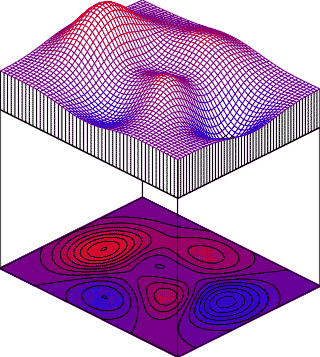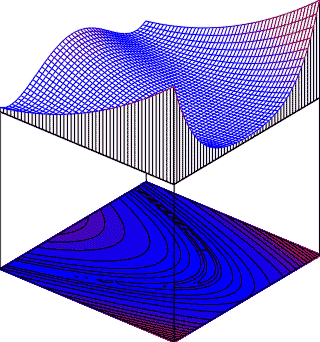##### The neural networks results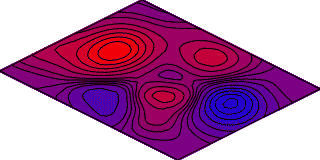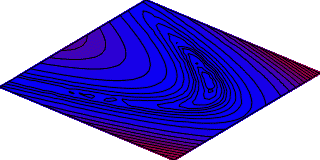Back...